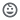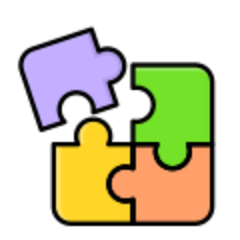# Tebak Angka & Matematika

50+Everyone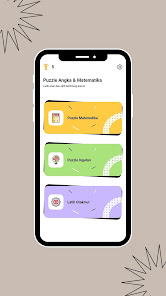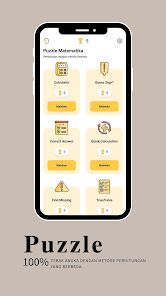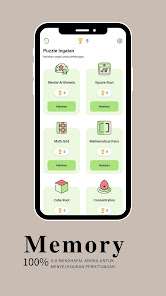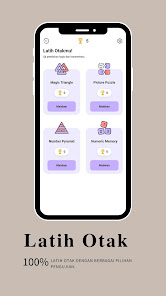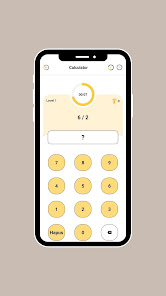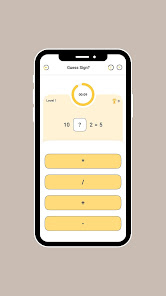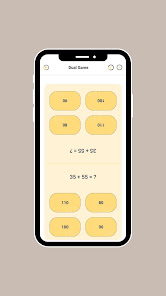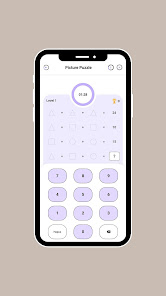Guess Numbers & Math is an app that has simple math games to learn and improve players math skills. This math game is easy and can be played by all age groups from children to adults.

This game has many modes that challenge the player to complete the game from levels 1-30. Each game session has a time limit and points scoring rules that will be included in the player's achievement statistics.

Calculator, solve the given equation correctly. Collect as many points as possible.
Guess Sign, find the correct arithmetic symbol to solve the given equation. Collect as many points as possible.
Square Root, find the square root of the given number. Collect as many points as possible.
Find Missing, find the correct number to solve the given equation. Collect as many points as possible.
True/False, select the correct statement to solve the given equation. Collect as many points as possible.
Dual Game, solve the given equation correctly and collect as many points as possible to beat the opponent.
Complex Calculation, find the correct sign to solve the given equation. Collect as many points as possible.
Cube Root, Numeric Memory, and Concentration challenge players to choose the correct number to solve the given equation. Collect as many points as possible.
Mathematical Pairs, each card contains equations and answers. Find and match the equation with the correct answer. Collect as many points as possible.
Correct Answer, select the correct number to solve the given equation. Collect as many points as possible.
Magic Triangle, the sum of each side of the triangle must be equal to the given number. To place the numbers, select the triangle circle and press the given number from the panel.
Mental Arithmetic, numbers with operators will be displayed. Remember the number with the operator and write the final answer. Collect as many points as possible.
Quick Calculation, solve simple equations. The faster it is solved, the more time will be given to solve the next equation.
Math Grid, select any number from the math grid to reach the answer shown above.
Picture Puzzle, each shape represents a number. Find the sum of each form of the given equation and solve the last equation.
Number Pyramid, the number of consecutive cells must be placed in the top cell. Fill in all cells correctly to complete the pyramid of numbers.
Updated on
Jul 29, 2022

## Data safety

Safety starts with understanding how developers collect and share your data. Data privacy and security practices may vary based on your use, region, and age. The developer provided this information and may update it over time.No data shared with third parties